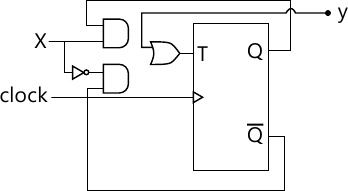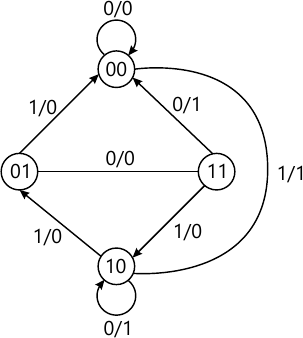Total marks: --
Total time: --
INSTRUCTIONS
(1) Assume appropriate data and state your reasons
(2) Marks are given to the right of every question
(3) Draw neat diagrams wherever necessary

1(a) Design a combinational circuit which takes two,2-bit binary numbers as its input and generates an output equal to 1, when the sum of the two numbers is odd.
6 M
1(b) Convert the given boolean function into:
i) R=f(a,b.c) =$(a^{-}-b)\left ( b+c^{-}\right )$
ii)$(a^{-}-b)\left ( b+c^{-}\right )$ Minterm canonical form
ii) p=f(x,y,z)=x+$=x+\overline{xy}(y+\overline z)$
6 M
1(c) Distinguish prime implicant and essential prime implicant. Determine PI and EPI for the given function N-f(a,b,c,d)-π (0,1,4,5,8,9,11)+d(2,10),Simplify the given function and implement using logic gates.
8 M

2(a) Simplify the given Boolean function using Quine-McCluskey method;
Y=f(a,b,c,d)=∑(01,2,6,7,9,10,12)+d(3,5).Verify the result using k-map.
10 M
2(b) Find the minimal sum and product for the given Boolean function,using MEV technique : Solve by using 3-variable map and 2-variable map y=f(a,b,c,d)-&Sigma(2,3,4,5,13,15)-8,9,10,11).
10 M

3(a) Distinguish between a decoder and an encoder.Implement full adder using IC 74138.
8 M
3(b) Implement 3- bit binary a gray code conversion by IC74139.
6 M
3(c) Design a priority encoder for a system with a 3 inputs,the middle bit with highest priority encoding to 10,the MSB with next priority encoding to 11, while the LSB with least priority encoding to 01.
6 M

4(a) Realize the following Boolean function : p=f(w,x,y,z)-&sigma(0,1,5,6,7,10,15)using :
i) 16 to 1MUX ii) 8:1 MUX iii) 4:1 MUX
10 M
10 M

5(a) explain the working of a master-slave SR flip-flop with the help of a logic diagram, function table,logic symbol and timming diagram.
10 M
5(b) With a neat logic diagram,explain the working of positive edge triggered d flip-flop.
10 M

6(a) Obtain the characteristic equation for D and T flip-flop.
6 M
6(b) With a neat logic diagram,explain the operation of 4-bit SISO unidirectional shift register.
6 M
6(c) Explain the working of four-bit binary ripple up counter,configured using positive edge triggered flip-flop. Also draw the timing diagram.
8 M

7(a) Design synchronous mod-6 counter using D flip - flop to generate the sequence 0,2,3,6,5,1,0......
10 M
7(b) Compare Mealy and Moore sequential circuit models.
4 M
7(c) Analyze the sequential circuit shown in fig. q7(c).
write input and output equations table,static table and static diagram.6 M

8(a) Write the basic recommended steps for the design of a clock synchronous sequential circuit.
6 M
8(b) Compare synchronous and asynchronous counter.
4 M
8(c) A sequential circuit has one input and one output.The state diagram is as shown in the fig.q8(c). design the sequential circuit with j-k flip - flop10 M

More question papers from Logic Design